# Easiest colleges to get into in Vermont

Top 7 colleges in Vermont with the highest acceptance rates
What are the easiest colleges to get into in Vermont? We've got you covered. We've compiled a national college database and have created a list of the easiest colleges to get into in Vermont below. These are the easiest 4 year colleges to get into in Vermont that have the highest acceptance rates, and are more likely to accept low GPAs, GEDs, and everything in between. There are tons of good colleges below that accept more applicants, and we also share their average SAT and ACT scores so that you can see where you're most competitive. Read on to find out more.

## Saint Michael's College acceptance rate

Saint Michael's College acceptance rate is 85.1%.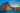The average SAT score for Saint Michael's College is 1231.

• SAT composite: 1231
• SAT math: 610

The average ACT score for Saint Michael's College is 27.

## Castleton State College of Vermont acceptance rate

Castleton State College of Vermont acceptance rate is 77.9%.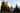The average SAT score for Castleton State College of Vermont is 1040.

• SAT composite: 1040
• SAT math: 510

The average ACT score for Castleton State College of Vermont is 20.

## Champlain College acceptance rate

Champlain College acceptance rate is 75.4%.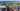The average SAT score for Champlain College is 1185.

• SAT composite: 1185
• SAT math: 580

The average ACT score for Champlain College is 25.

## University of Vermont acceptance rate

University of Vermont acceptance rate is 67.3%.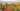The average SAT score for University of Vermont is 1270.

• SAT composite: 1270
• SAT math: 630

The average ACT score for University of Vermont is 29.

## Norwich University acceptance rate

Norwich University acceptance rate is 65.7%.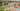The average SAT score for Norwich University is 1130.

• SAT composite: 1130
• SAT math: 560

The average ACT score for Norwich University is 23.

## Bennington College acceptance rate

Bennington College acceptance rate is 56.6%.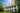The average SAT score for Bennington College is 1340.

• SAT composite: 1340
• SAT math: 640

The average ACT score for Bennington College is 29.

## Middlebury College acceptance rate

Middlebury College acceptance rate is 15.4%.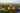The average SAT score for Middlebury College is 1445.

• SAT composite: 1445
• SAT math: 735